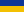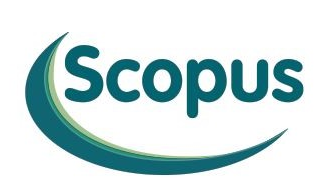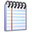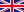Asymptotic estimates for the solutions of boundary-value problems with initial jump for linear differential equations with small parameter in the coefficients of derivatives / Kasymov, K. A., Nurgabyl, D. N., Uaisov, A. B. (2013)web address of the page http://jnas.nbuv.gov.ua/article/UJRN-0000790631  Ukrainian Mathematical JournalА - 2018  /Issue (2013, Т. 65, № 5)Касымов К. А., Нургабыл Д. Н., Уаисов А. Б.Асимптотические оценки решения краевой задачи с начальным скачком для линейных дифференциальных уравнений с малым параметром при производных Бібліографічний опис:Касымов К. А., Нургабыл Д. Н., Уаисов А. Б. Асимптотические оценки решения краевой задачи с начальным скачком для линейных дифференциальных уравнений с малым параметром при производных. Український математичний журнал. 2013. Т. 65, № 5. С. 629–641. URL: http://jnas.nbuv.gov.ua/article/UJRN-0000790631Ukrainian Mathematical Journal   /Issue (2013, 65 (5))Kasymov K. A., Nurgabyl D. N., Uaisov A. B.Asymptotic estimates for the solutions of boundary-value problems with initial jump for linear differential equations with small parameter in the coefficients of derivativesCite:Kasymov, K. A., Nurgabyl, D. N., Uaisov, A. B. (2013). Asymptotic estimates for the solutions of boundary-value problems with initial jump for linear differential equations with small parameter in the coefficients of derivatives. Ukrainian Mathematical Journal, 65 (5), 629–641. http://jnas.nbuv.gov.ua/article/UJRN-0000790631 [In Russian].

 National Academy of Sciences of UkraineLibrary portal of National Academy of Sciences of Ukraine | LibNAShttp://libnas.nbuv.gov.uaVernadsky National Library of Ukraine (VNLU)Institute of Information Technologies of VNLU+38 (044) 525-36-24libnas@nbuv.gov.uaUkraine, 03039, Kyiv, Holosiivskyi Ave, 3, room 209